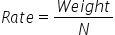Mathematics
Easy

Question

# The total weight of 4 tyres is 800 pounds. ___________ pounds would be the weight of 7 tyres, at the same rate.

## 90010001400850Hint:

## The correct answer is: 1400

### The weight of 4 tyres is 800 pounds.Let the number of tyres be denoted as N.We have to find the weight of the 7 tyres.Let’s find the rate by taking the ratio of weight and the number of tyres. From the ratio, we will find the weight per tyre. And we will use this to find the weight of 7 tyres.As rate is constant, using this value we will find the weight of 7 tyresWe will rearrange the expression for weight by multiplying both the sides by N.Rate × N = weightWeight = Rate × N= 200 × 7= 1400 poundsTherefore, the weight of 7 tyres is 1400 pounds.

The other method is to take the ratios and directly equate them. As the rate is constant, so the ratios will be constant. So we will consider the quantity we have to find as x and then equate the ratios. It will be a shortcut.

### Related Questions to study#### With Turito Foundation.#### Get an Expert Advice From Turito.